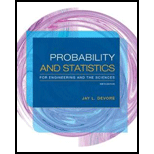Chapter 3, Problem 122SE### Probability and Statistics for Eng...

9th Edition
Jay L. Devore
ISBN: 9781305251809

#### Solutions

Chapter
Section### Probability and Statistics for Eng...

9th Edition
Jay L. Devore
ISBN: 9781305251809
Textbook Problem

# Consider a communication source that transmits packets containing digitized speech. After each transmission, the receiver sends a message indicating whether the transmission was successful or unsuccessful. If a transmission is unsuccessful, the packet is re-sent. Suppose a voice packet can be transmitted a maximum of 10 times. Assuming that the results of successive transmissions are independent of one another and that the probability of any particular transmission being successful is p, determine the probability mass function of the rv X = the number of times a packet is transmitted. Then obtain an expression for the expected number of times a packet is transmitted.

To determine

Find the pmf of X.

Find the expression for the expected number of times a packet is transmitted.

Explanation

Given info:

A communication source transmits packets containing digitized speech. The receivers sends a message whether the transmission was successful or not after each transmission. If it is unsuccessful, the packet is re-sent. A packet can be re transmitted maximum 10 times.

X be the number of times a packet is transmitted.

Calculation:

The pmf of X:

The all possible values of x are 1,2…10.

The probability of getting x times transmitted is 1st (x – 1) times failed, at xth time it was completed.

Thus,

P(X=x)=(1p)x1p

If x = 10, then P(X=10)=(1p)9

Hence, the pmf is

P(X=x)={(1p)x1p,x=1,2.

### Still sussing out bartleby?

Check out a sample textbook solution.

See a sample solution

#### The Solution to Your Study Problems

Bartleby provides explanations to thousands of textbook problems written by our experts, many with advanced degrees!

Get Started

## Additional Math Solutions

#### Define Web Services.

Accounting Information Systems

#### In Exercises 73-80, find the indicated limits, if they exist. 75. limx3x3+x2+1x3+1

Applied Calculus for the Managerial, Life, and Social Sciences: A Brief Approach

#### 8. If find and .

Mathematical Applications for the Management, Life, and Social Sciences

#### Proof Prove that if limx0f(c+x)=f(c) then f is continuous at c.

Calculus: Early Transcendental Functions (MindTap Course List)